# Lesson 9 Logical Operators

In this lesson, we will learn about the logical operators, AND, OR and NOT and we are going to see that how do we change a simple Boolean statement into the complex statements that have multiple components.

So this lesson is linked with the previous lesson in which we have given a detailed overview about Boolean operation and equality and inequality in JavaScript, so still, we are going to evaluate true or false throughout this lesson but there might be multiple pieces that individually evaluate to be true or false.

Logical operators are mainly used to determine the logics between variables and values.
So there are three logical operators

1. AND
2. OR
3. NOT

## Logical Operators

AND, OR, and NOT

Operator Name Example Result
&& AND A < 20 && A !== 10 false
｜｜ OR B > 18 A === 10
! NOT !(A === B) true

Assume A=10 and B=18

## AND Operator:

AND operator represents with && and it takes two sides: the right side and the left side and, AND operator goes to the middle as you can see in above table.

`A < 20 && A !== 10`

So the way to read this is A is less than 20 AND A is not equals to 10. So if we evaluate both sides, then A is less than 20 that is true,

A < 20 (this statement is true)
But A is not equal to 10, it is false,
A !== 10 (this statement is False)

Because we are assuming A=10 and B=18. So the result is false.

And the way AND statement works, it requires both sides to be matched.
In our case one side is true but another is false, so the whole statement is false.

## OR Operator:

OR statement is slightly different from AND statement.
OR Operator represents with double vertical bar (||).
It also takes both side statement i-e: right and left but it requires only one side statement, whether it is on left or right, to be true in order to make the complete statement true.

Here is an example of OR statement in above table and it read as, B is greater than 18 OR A is triple equal to 10.

B > 18 || A === 10

You can notice there, it is a combination of two statement, one is on the left and another on the right.

B > 18 (which is not true)

Since B actual value is 18, that’s why it can’t be greater than 18.

A === 10 (which is actually true)

Here is A whose actual value is 10 is triple equal to 10.

So the result is true because one side represents the true statement.

## NOT Operator

NOT represents with exclamation character (!).
It does not take a right or left side, it just negates or flips the value of whatever we apply to it.
So if something was true but we put NOT there, it would be false and vice versa.

Let’s do a quick exercise, which we have covered so far in this lesson.

## Exercise

``````var A = 50;
var B = "z" ;
B ==  "x" || A >= 50;
``````

Its three lines of code, where A is 50, B is a string “z” and third one is an expression that uses OR in a middle.

So go ahead and evaluate this:

A is equal to 50 and B is equal to “z”, and expression says that

B is equal to string z which is FALSE OR

A is greater or equal to 50 which is TRUE.

So the result would be TRUE because is it using OR method.

Here is an output of above code.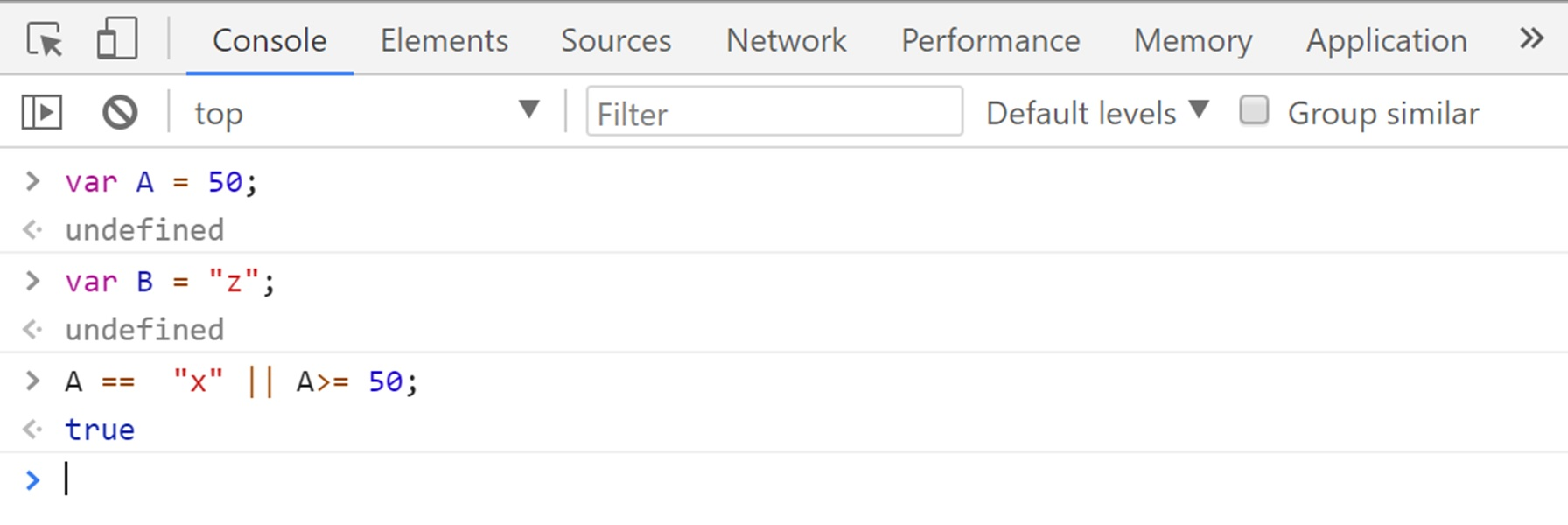## TRUTHY AND FALSY VALUES

Values that are not actually True or False, are still inherently “truthy” or “falsie” when evaluated in a Boolean context.

Every value in JavaScript is inherently Truthy or Falsie.
Aside from the obvious Boolean and True and False which are clearly true and false, every other value has this inherent truthiness and falseness that JavaScript assigns them.

So here is some example just to show you a way to finding this out.

## JavaScript Code

So what if I just want to know if the string “Welcome to my Lesson” is true or false, let see what happen if we just type the string and hit enter.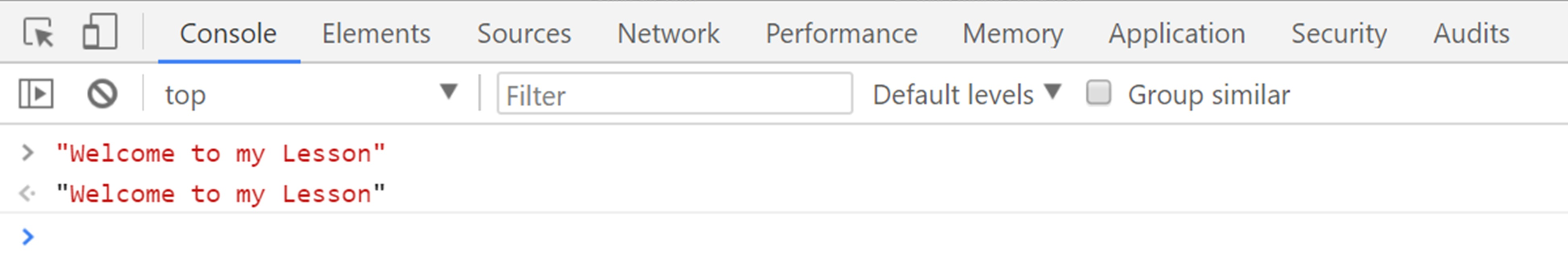You can see in above Screenshot when we write String and hit enter then it just gives me the exact string back.

Now let see what happened if we put it in a Boolean statement just to negate it, I typed !“Welcome to my Lesson”, and it gave me that it is false.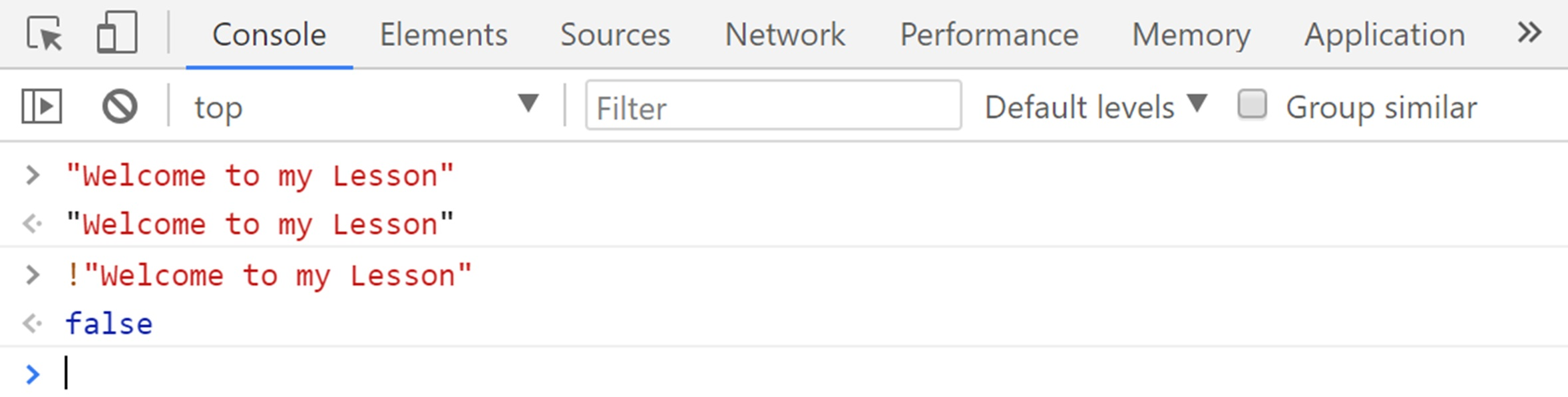It is because it indicated if we get false back that tells me that the string “Welcome to my Lesson” is truthy Whereas, when I double negated that “Welcome to my Lesson” with !!, it will flip it and then flip it back and tell me that the string is true because single negated string showed me the falsie.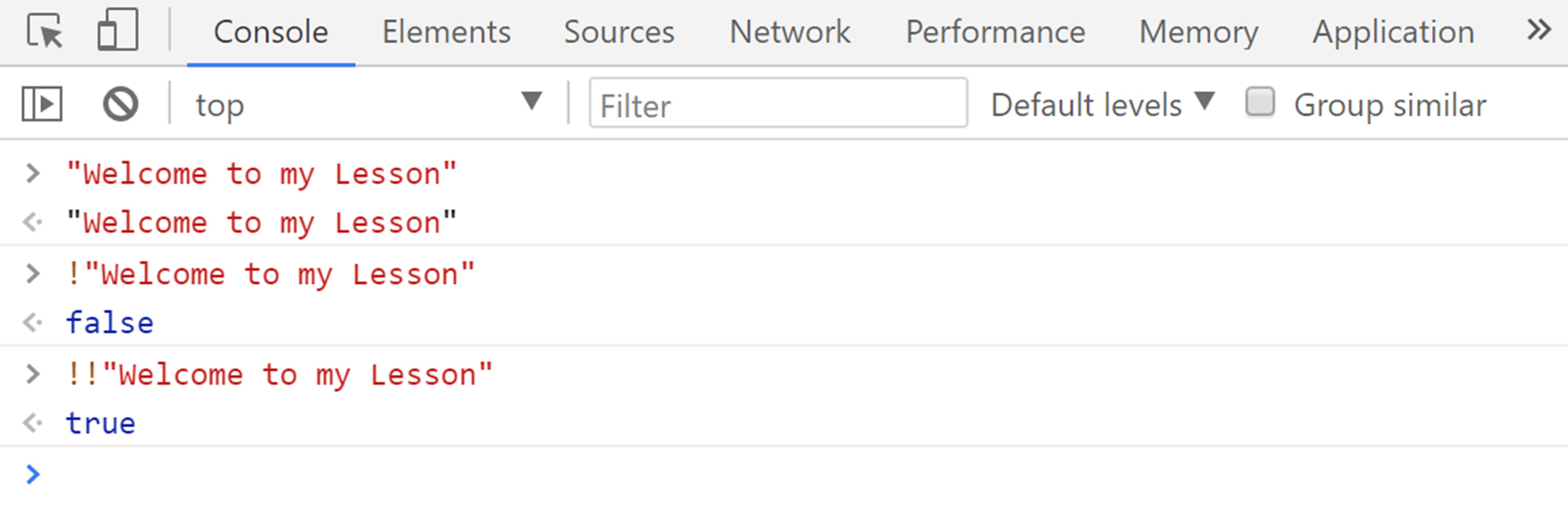And let see what happen when we ado the same thing on an empty string and null string, which are always false, it gave me false statement.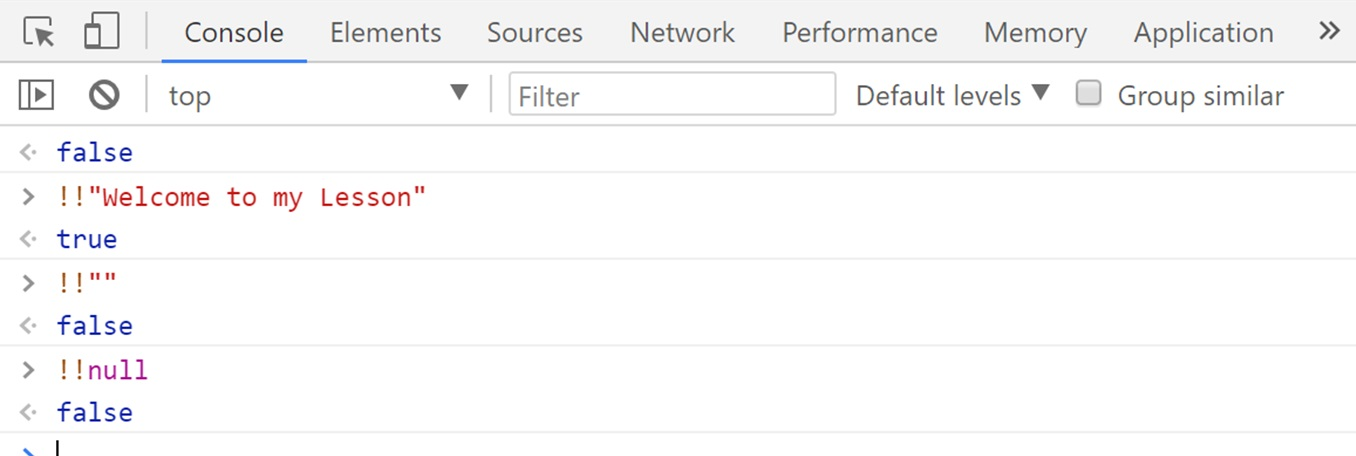So these are things that in some languages behave a little bit differently so just because in JavaScript !!null is falsie that does not mean that in other languages it is automatically falsie.

In this lesson, we learned about the logical operators, AND, OR and NOT and we have gone through simple Boolean statement and we have also learned that how we can solve complex Boolean expression.